• 在FPGA上实现单精度浮点加法器的设计,通过分析实数的IEEE 754表示形式和IEEE 754单精度浮点的存储格式,设计出一种适合在FPGA上实现单精度浮点加法运算的算法处理流程,依据此算法处理流程划分的各个处理模块便于流水...
• 舍入过程中可以使用直接choping和就近舍入，考虑可就近舍入过程中引起尾码加一导致阶码增加的情况。已通过Quartus_ii\Modelsim的联合仿真。
• 摘　要: 采用Verilog HDL语言, 在FPGA上实现了32位单精度浮点乘法器的设计, 通过采用改进型Booth编码，和Wallace 树结构, 提高了乘法器的速度。本文使用Altera Quartus II 4.1仿真软件, 采用的器件是EPF10K100EQ 240...
• 单精度浮点转换器用于把十进制，十六进制的单精度浮点相互转换
• S7-200SMART 双精度浮点型数据转换为单精度浮点型的方法
• 单精度浮点的表示范围：-3.40E+38 ~ +3.40E+38 双精度浮点的表示范围：-1.79E+308 ~ +1.79E+308 单精度实数在内存中占32bit 有效数字为6 ~ 7位，双精度实数占内存单元为64bit 有效数字为15 ~ 16位，没有说明时,实型...
1.在内存中占有的字节数不同 单精度浮点数占4个字节 双精度浮点数占8个字节
2.有效数字位数不同 单精度浮点数有效数字8位 双精度浮点数有效数字16位
3.所能表示数的范围不同 单精度浮点的表示范围：-3.40E+38 ~ +3.40E+38 双精度浮点的表示范围：-1.79E+308 ~ +1.79E+308  单精度实数在内存中占32bit 有效数字为6 ~ 7位，双精度实数占内存单元为64bit 有效数字为15 ~ 16位，没有说明时,实型常量是作为双精度处理的,若要使用单精度需在后面加上字母f或F,如111.222f… double能够表示的小数点的位数更多，更精确.
展开全文java
• 实际上，任何精度浮点运算也可以像BigInteger那样计算，可以用多个浮点数据表示更高精度浮点数据。而这就要求实现带余数的浮点四则运算。 带余数的浮点四则运算的定义： 加法和减法，余数和结果的和能精确表示...
实际上，任何精度的浮点运算也可以像BigInteger那样计算，可以用多个浮点数据表示更高精度的浮点数据。而这就要求实现带余数的浮点四则运算。
带余数的浮点四则运算的定义：
加法和减法，余数和结果的和能精确表示运算值。
乘法和除法，余数和结果必须同号，对于乘法，余数和结果的和能精确表示运算值，而对于除法，余数就是原来的意思。

不多解释，代码如下：（修复某些可能存在错误）
#include <iostream>
#include <xmmintrin.h>

using namespace std;

typedef struct {
float hi;
float lo;
} doublf;

/**
* BigFloat的实际值为：
* 使用这个数据类型的缺点是数据存储冗余，优点是便于计算。
**/
typedef struct {
int32_t len;//data中有效数据的长度
int32_t exp;//指数部分
float data;//浮点数据，每个都是1.x的数据
} BigFloat;

inline int32_t ifabs(float a){
int32_t p=*(int32_t*)&a;
return p&0x7fffffff;
}

//o=5
doublf ret;
ret.hi=a+b;
if(ifabs(a)>ifabs(b))ret.lo=b-(ret.hi-a);
else ret.lo=a-(ret.hi-b);
return ret;
}

//o=5
doublf subf(float a,float b){//带余数减法
doublf ret;
ret.hi=a-b;
if(ifabs(a)>ifabs(b))ret.lo=(a-ret.hi)-b;
else ret.lo=a-(ret.hi+b);
return ret;
}

//o=14
doublf mule(float a,float b){
doublf ret;
float m,n;//结合整数运算优化
int32_t ap,bp,am,bm;
uint32_t mp;
ap=*(int32_t*)&a;
bp=*(int32_t*)&b;
ret.hi=n=a*b;
am=ap&0xff800000;
bm=bp&0xff800000;
am=am+bm-0x4b000000;
m=*(float*)&am;
ap|=0x800000;
bp|=0x800000;
mp=(uint32_t)ap*(uint32_t)bp;
ap=(mp&0x7fffff)|am;
ret.lo=*(float*)&ap;
if(mp&0x800000){
if(n+m!=n)ret.lo-=m;
}
else ret.lo-=m;
return ret;
}

//o=14
doublf mulf(float a,float b){//带余数乘法
unsigned int csr=_mm_getcsr();
doublf ret=mule(a,b);
_mm_setcsr(csr);
return ret;
}

//o=17
doublf divf(float a,float b){//带余数除法
unsigned int csr=_mm_getcsr();
doublf ret,tmp;
ret.hi=a/b;
tmp=mule(ret.hi,b);
ret.lo=(a-tmp.hi)-tmp.lo;
_mm_setcsr(csr);
return ret;
}

//检验正确
//o=20,8
doublf ret,tmp;
/* 计算 */
if(ifabs(a.hi)<ifabs(b.hi)){
tmp=a;
a=b;
b=tmp;
}
ret.hi=tmp.hi;
ret.lo+=tmp.lo;
ret.hi=tmp.hi;
ret.lo+=tmp.lo;//即使高低位重叠也不会有影响
return ret;
}

//检验正确
//o=22,9
doublf sub(doublf a,doublf b){//最後一位会产生误差
doublf tmp;
/* 计算 */
tmp.hi=-b.hi;
tmp.lo=-b.lo;
}

//检验正确
doublf mul(doublf a,doublf b){
#if 0//最後一位会产生误差
//o=137,25
unsigned int csr=_mm_getcsr();
doublf ret,tmp,tmp2,tmp3,tmp4;
/* 计算 */
tmp=mule(a.lo,b.lo);
tmp2=mule(a.lo,b.hi);
tmp3=mule(a.hi,b.lo);
tmp4=mule(a.hi,b.hi);
_mm_setcsr(csr);
return ret;
#else//最後两位会产生误差
//o=25,8
doublf ret=mulf(a.hi,b.hi);
ret.lo+=(a.lo*b.lo+a.lo*b.hi)+a.hi*b.lo;
return ret;
#endif
}

//检验正确
doublf div(doublf a,doublf b){//最後两位会产生误差
#if 1//o=27,10
doublf ret,tmp;
float d=b.hi+b.lo;
ret.hi=a.hi/d;
unsigned int csr=_mm_getcsr();
/* 计算 */
tmp=mule(b.hi,ret.hi);
//tmp2=mule(b.lo,ret.hi);
_mm_setcsr(csr);
ret.lo=((((a.hi-tmp.hi)-tmp.lo)-ret.hi*b.lo)+a.lo)/d;//精度低但更快
//a=sub(a,tmp);
//a=sub(a,tmp2);
//ret.lo=a.hi/d;
return ret;
#else//o=24,8
doublf ret;
float d=b.hi+b.lo;
ret=divf(a.hi,d);
ret.lo=(ret.lo-ret.hi*((d-b.hi)-b.lo)+a.lo)/d;
return ret;
#endif
}

inline double to_double(doublf a){
return (double)a.hi+a.lo;
}

inline doublf to_doublf(double a){
doublf ret;
ret.hi=a;
ret.lo=a-ret.hi;
return ret;
}

/**
* 这样就能有办法用单精度模拟双精度（可以有2^-46的精度），甚至模拟任何精度的浮点数据。
* 关键是要支持带余数的浮点四则运算。
**/

int main()
{
int i;
uint64_t tmp;
doublf df,af,tf;
do{
tf=df=to_doublf(dd);
cin>>i;
while(i--){
tmp=*(uint64_t*)&tt;
tmp=*(uint64_t*)&td;
cout<<"chk="<<hex<<tmp<<endl;
dd=tt;
};
df=tf;
dd=to_double(tf);
cin>>i;
while(i--){
tt=to_double(df=sub(df,af));
tmp=*(uint64_t*)&tt;
cout<<"sub="<<hex<<tmp<<endl;
tmp=*(uint64_t*)&td;
cout<<"chk="<<hex<<tmp<<endl;
dd=tt;
};
df=tf;
dd=to_double(tf);
cin>>i;
while(i--){
tt=to_double(df=mul(df,af));
tmp=*(uint64_t*)&tt;
cout<<"mul="<<hex<<tmp<<endl;
tmp=*(uint64_t*)&td;
cout<<"chk="<<hex<<tmp<<endl;
dd=tt;
};
df=tf;
dd=to_double(tf);
cin>>i;
while(i--){
tt=to_double(df=div(df,af));
tmp=*(uint64_t*)&tt;
cout<<"div="<<hex<<tmp<<endl;
tmp=*(uint64_t*)&td;
cout<<"chk="<<hex<<tmp<<endl;
dd=tt;
};
cin>>dd;
return 0;
}


展开全文算法 c++
• 该工具为单精度与双精度的浮点转换成十进制，或者十进制转换为单精度或双精度的工具，可以验证你的计算方法是否错误。
• 目录1.实现目标2.原理说明3.设计说明4.Verilog代码 1.实现目标 2.原理说明 首先要懂得浮点乘法器的工作原理与如何编码运算的过程，这里给出两个参考Blog. 浮点加法、减法, 乘法、除法运算:...本次设计输入有两个32位


目录
1.实现目标2.原理说明3.设计说明4.Verilog代码

1.实现目标2.原理说明
首先要懂得浮点乘法器的工作原理与如何编码运算的过程，这里给出两个参考Blog. 浮点加法、减法, 乘法、除法运算: https://blog.csdn.net/xingqingly/article/details/18981671 计算机中定点数表示方法——移码: https://blog.csdn.net/Dnesity/article/details/104531681
3.设计说明
本次设计输入有两个32位单精度浮点数，时钟信号，复位信号，使能信号和round_cfg，输出有结果以及溢出标志； 考虑到阶码可能出现溢出，采用双符号位，将移码转为补码进行运算，再转回移码的策略判断溢出； 同时考虑到尾数为0的不规范的可能性，将特殊处理，将输出全部置为0. 变量类型为reg，无符号类型，但为了实现能进行有符号类型的计算，需要对输入的32位单精度浮点数进行提取.
4.Verilog代码
//**
//Author:                   Luk.wj
//Create:                  2020.11.19
//Revise:                  2020.11.24
//Fuction:   Two-stage assembly line floating point multiplier with single precision
//功能：              2级流水线的单精度浮点乘法器(IEEE Standard)
//**
module two_line_float_p_mul(flout_a,flout_b,clk,en,rst,round_cfg,flout_c,overflow);
input[31:0] flout_a;           // 输入的被乘数
input[31:0] flout_b;           // 输入的乘数
input clk;                     // 时钟信号
input en;                      // 使能信号
input rst;                     // 复位信号
input round_cfg;               // 决定舍入的方法，0采用chopping，1采用就近舍入
output[31:0] flout_c;          // 输出运算结果
output[1:0] overflow;          // 输出溢出标志
reg[31:0] flout_c;
reg[1:0] overflow;
reg s1,s2;                     // 输入数符号
reg[7:0] exp1,exp2;            // 输入阶码
reg[23:0] man1,man2;           // 输入尾数，多一位，把默认的‘1’加上
reg n;                         // 左归阶码
reg[9:0] temp1,temp2,temp3;    // 多两位，用于阶码的双符号表示，判断溢出
reg[47:0] mul_out_p;           // 第二级逻辑运算尾数部分

//-------‘s'为符号，'e'为阶码，'m’为尾数------------//
//第一级逻辑输出
reg       one_s_out;
reg[9:0]  one_e_out;
reg[47:0] one_m_out;

//第一级流水寄存
reg       one_s_reg;
reg[9:0]  one_e_reg;
reg[47:0] one_m_reg;

//第二级逻辑输出
reg[1:0]  two_f_out; //溢出
reg[7:0]  two_e_out;
reg[22:0] two_m_out;

//第二级流水寄存
reg       two_s_reg;
reg[1:0]  two_f_reg; //溢出
reg[7:0]  two_e_reg;
reg[22:0] two_m_reg;

/*---------------提取flout_a 的符号,阶码,尾数---------------------*/
always@(*)
begin
if(!rst)
begin       //复位，初始化
s1   = 1'b0;
exp1 = 8'b0000_0000;
man1 = {1'b1,23'b0};
end
else if(en)
begin
s1   = flout_a;
exp1 = flout_a[30:23];
man1 = {1'b1,flout_a[22:0]};
end
end

/*---------------提取flout_b 的符号,阶码,尾数---------------------*/
always@(*)
begin
if(!rst)
begin      //复位，初始化
s2   = 1'b0;
exp2 = 8'b0000_0000;
man2 = {1'b1,23'b0};
end
else if(en)
begin
s2   = flout_b;
exp2 = flout_b[30:23];
man2 = {1'b1,flout_b[22:0]};
end
end

/*--------------------第一级逻辑运算---------------------------------*/
//符号位
always@(*)  one_s_out = s1^s2;  //输入符号异或

//尾数相乘
always@(*)
begin
if(man1 == 24'b10000000000_0000000000000)
one_m_out = 48'b0;
else if(man2 == 24'b10000000000_0000000000000)
one_m_out = 48'b0;
else
one_m_out = man1*man2; //48位
end

//阶码相加,阶码是移码,移码是符号位取反的补码
always@(*)
//把阶码的移码形式变为补码形式，并且转成双符号位格式,00为正,11为负
begin
if(exp1 == 1)
temp1 = {2'b00,1'b0,exp1[6:0]};
else
temp1 = {2'b11,1'b1,exp1[6:0]};
if(exp2 == 1)
temp2 = {2'b00,1'b0,exp2[6:0]};
else
temp2 = {2'b11,1'b1,exp2[6:0]};
one_e_out[9:0] = temp1[9:0] + temp2[9:0];        //阶码以双符号补码的形式相加计算
end
/*----------------------------------------------------------------*/

//第一级流水寄存
always@(posedge clk)
begin
one_s_reg <= one_s_out;
one_e_reg <= one_e_out;
one_m_reg <= one_m_out;
end

/*--------------------第二级逻辑运算---------------------------------*/
//尾数规范化及舍入处理，溢出判断
always@(*)
begin
if(one_m_reg == 48'b0000000000_0000000000000)
begin
two_m_out =  23'b0;
n  =  1'b0;
end  // 处理特殊值
else
begin
if(one_m_reg == 1)
begin
n  = 1'b1;                  //左归码为1
mul_out_p = one_m_reg >> 1; //右移一位
end
else
begin
n   = 1'b0;                 //左归码为0
mul_out_p = one_m_reg;      //不需要右移
end
if(round_cfg == 1)         //0采用chopping，1采用就近舍入
begin
if(mul_out_p == 1)
two_m_out[22:0] = mul_out_p[45:23]+1'b1;
else
two_m_out[22:0] = mul_out_p[45:23];
end
else
two_m_out[22:0] = mul_out_p[45:23];
end
//双符号的定义，01为上溢，10为下溢，符号相同无溢出
temp3 = one_e_reg[9:0] + n + 1'b1;  //加上左归阶码,因为补码与移码的转换是-128，而IEEE是-127，故加上1
if(temp3[9:8] == 2'b01)
two_f_out = 2'b01; //阶码上溢
else if(temp3[9:8] == 2'b10)
two_f_out = 2'b10; //阶码下溢
else
two_f_out = 2'b00; //无溢出
//输出补码转回移码
case(temp3)
1'b1:  two_e_out = {1'b0,temp3[6:0]};
1'b0:  two_e_out = {1'b1,temp3[6:0]};
endcase
end
/*----------------------------------------------------------------*/

//第二级流水寄存
always@(posedge clk)
begin
if(two_m_out == 0) //特殊值处理
begin
two_s_reg <= 1'b0;
two_e_reg <= 8'b0;
two_m_reg <= 23'b0;
two_f_reg <= 2'b00;
end
else
begin
two_s_reg <= one_s_reg;
two_f_reg <= two_f_out;
two_e_reg <= two_e_out;
two_m_reg <= two_m_out;
end
end

//输出结果
always@(*)
begin
flout_c  = {two_s_reg,two_e_reg[7:0],two_m_reg[22:0]};
overflow = two_f_reg;
end

endmodule


展开全文verilog
• 小数部分乘2整数部分是0就写0，不是0就写1，乘完结果小数部分是0就结束，或者设置一个精度精度到几位就用小数部分乘多少位 小数部分，不设精度永远算不完 小数点移位 保证左边只有一个 1 0.25转换为二进制例子 ...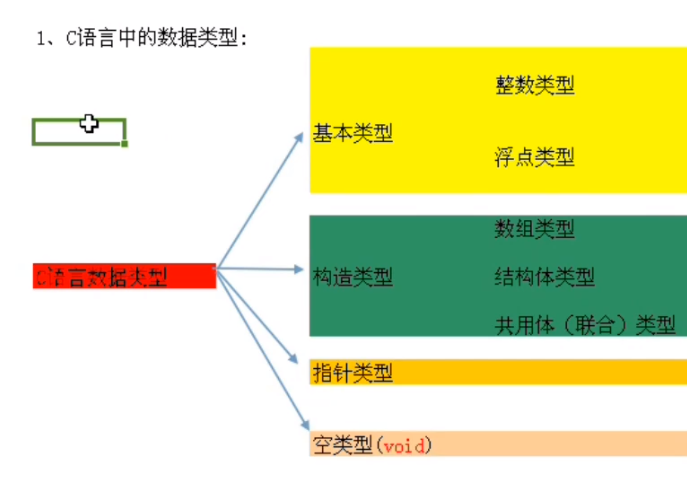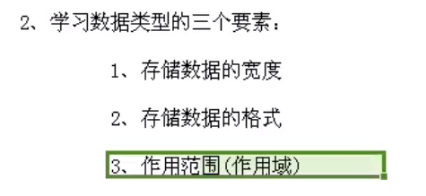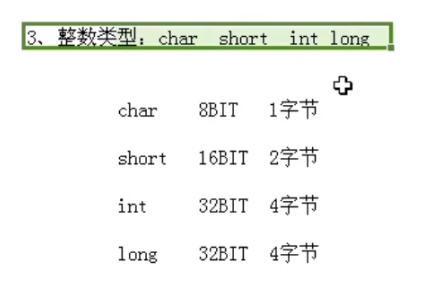什么都不写默认是有符号的，如果只写unsigned 或signed 默认是int 类型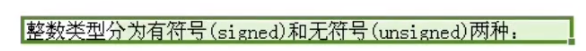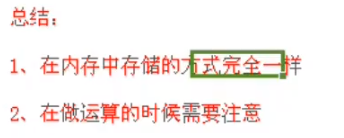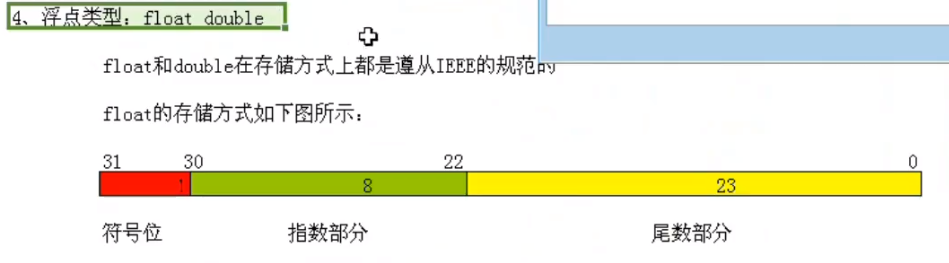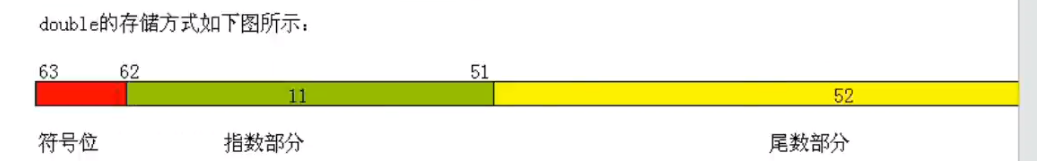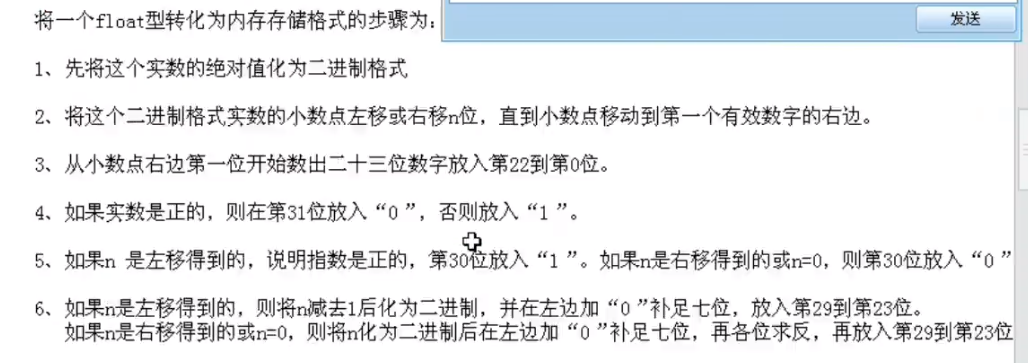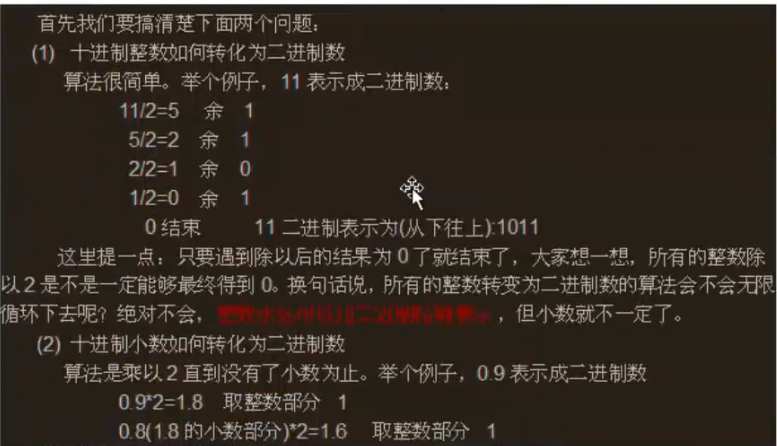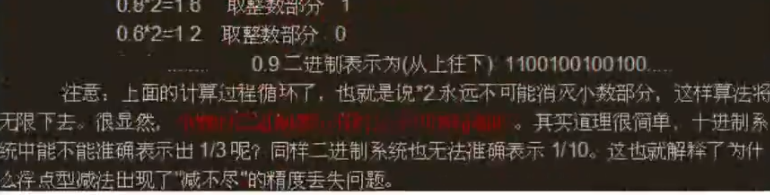浮点数转进制
整数部分除2余数是0就写0 ，不是0就写1，除完整数部分是0就结束
小数部分乘2整数部分是0就写0，不是0就写1，乘完结果小数部分是0就结束，或者设置一个精度，精度到几位就用小数部分乘多少位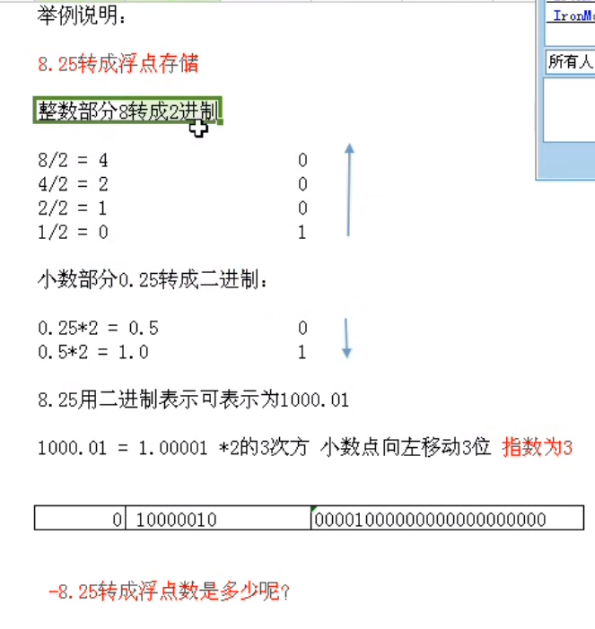小数部分，不设精度永远算不完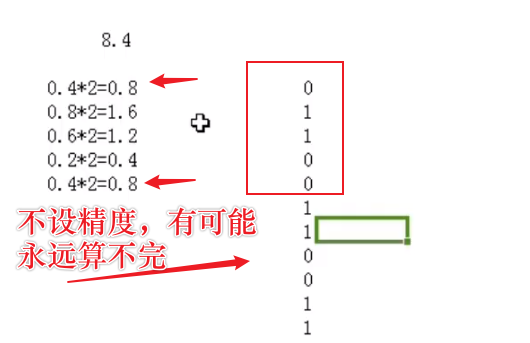小数点移位 保证左边只有一个 1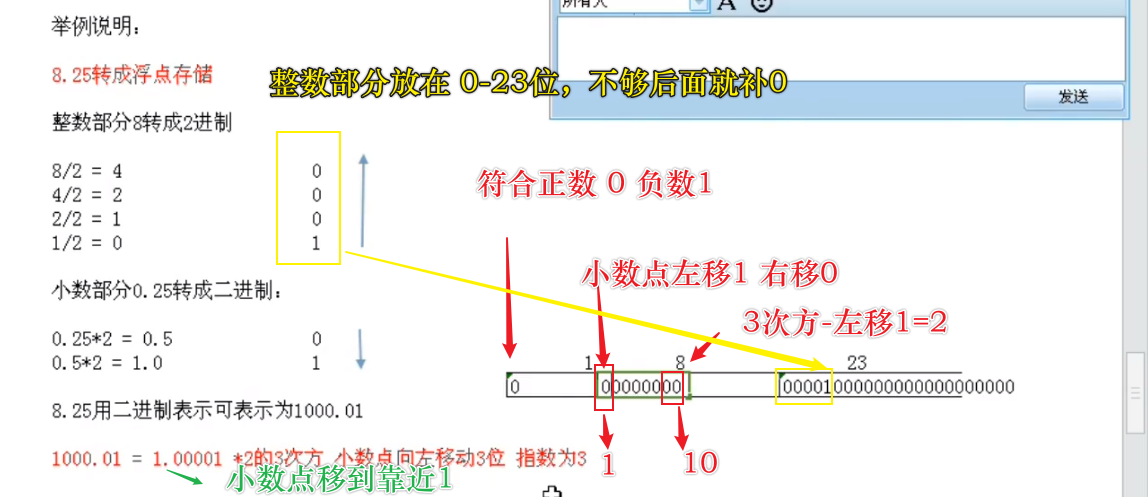0.25转换为二进制例子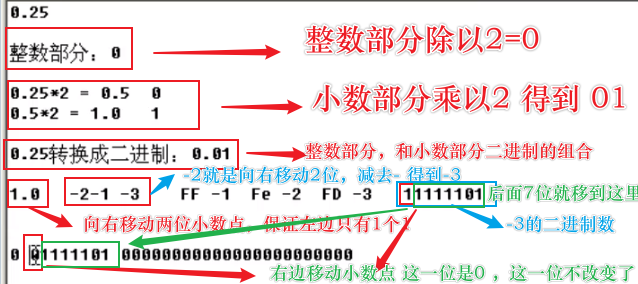转为 16进制的结果C语言加返汇编测试
#include <stdio.h>

void Plus()
{
float x = 0.25f;

}

int main(int argc,char* argv[])
{

Plus();

return 0;
}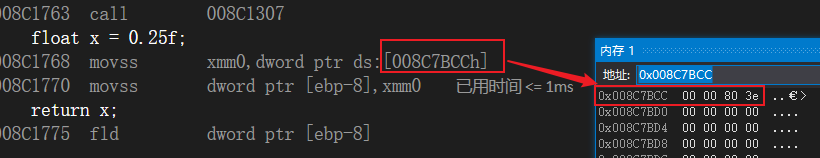另外一种更加简单10进制浮点转二进制的方法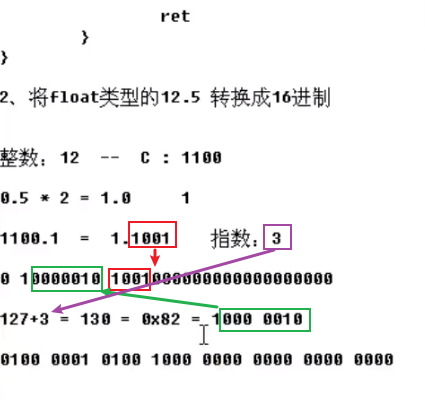展开全文• Variant转换成Byte数组，单精度浮点型数据转换为16进制的字符串，并进行大端转小端变换，字符串转换成Byte数组，BYTE型数据转换为String型数据，四字节16进制字符串转换为32位单精度浮点型，并大端模式转为小端模式...
• 1、操作数预处理 begin rA <= { A, A[30:23], 1’b1, A[22:0] }; rB <= { B, B[30:23], 1’b1, B[22:0] }; end 2、运算 begin isSign <= A ^ B; BDiff = rB[31:24] - 8...= rA[31:24] - B...
• 首先，float单精度浮点型，计算机会分配4字节，32位来进行存储，而double float双精度浮点型，计算机则会分配8个字节，即64位来进行小数的存储，这是后面进行详细解释的必备知识。 其次，计算机在存储浮点型数据时，...
• 整型转单精度浮点 指定的 32 位整数重新解释为单精度浮点值 .netFrameWork 自定义函数 //ModbusRTU格式的2个32位整数转浮点数 public float parseInt2Float(int x1, int x2) { int f, fRest, exponent, ...c#
• 深入理解如何进行单精度浮点计算，怎样才能使计算出来的误差更小。
• 浮点运算单元FPU（Floating-point Unit）在当前CPU的运算中地位越来越重要，论文中实现了一种基于FPGA的快速单精度浮点运算器。该运算器采用了流水线和并行计算技术，使得浮点数运算的速度有了显著的提高。在QUARTUS...
• ## IEEE 754标准 单精度浮点规格化

千次阅读 多人点赞 2019-12-25 20:53:31
顾名思义，是IEEE 协会制定的一个标准，将规格化浮点数分为单精度(32位)、双精度(64位)、扩展精度(80位) 单精度 符号位(31) 阶码(30-23) 尾数有效位(22-0) 如上所示，符号位占1位，阶码占8位，尾数占23位。 ...
• 关于16进制浮点数对于大小为32-bit的浮点数（32-bit为单精度，64-bit浮点数为双精度，80-bit为扩展精度浮点数），1、其第31 bit为符号位，为0则表示正数，反之为复数，其读数值用s表示；2、第30～23 bit为幂数，其读...
• 在CUDA 中如果不指明是 单精度， CUDA会调用双精度实现。 （血泪呀！！！） 如果要使用单精度，要指明， 即使用 形如__fmul_rn(x,y)的函数。 详见链接！！ ...
• 32位浮点ALU 32位浮点单元，用于执行加法和减法。VHDL
• 最近在搞DSP，所以不可避免地会遇到浮点数，包括半精度浮点(16bit) 和单精度浮点(32bit)。 1、根据wiki百科介绍，IEEE 754规范中规定的32bit float point的格式为：（以下引用wiki百科......